Charged Particles in Uniform Electric Fields Forces and

• Slides: 14Charged Particles in Uniform Electric Fields Forces and Fields 9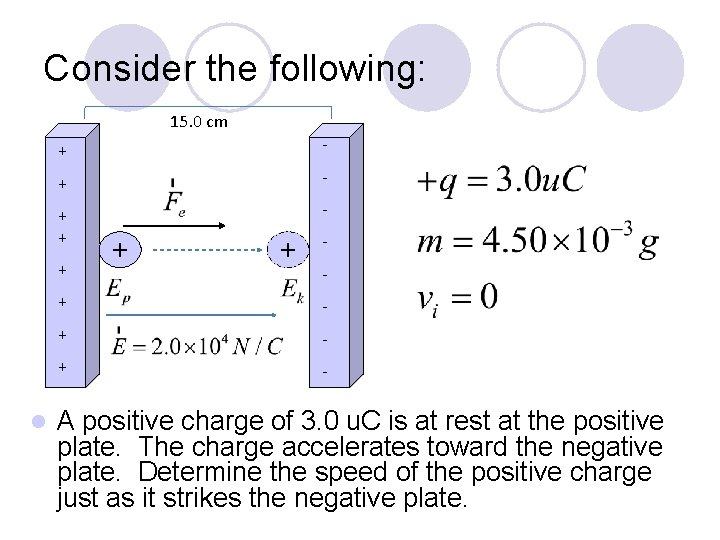Consider the following: 15. 0 cm + - + l + + - + - A positive charge of 3. 0 u. C is at rest at the positive plate. The charge accelerates toward the negative plate. Determine the speed of the positive charge just as it strikes the negative plate.1. Kinematics Solution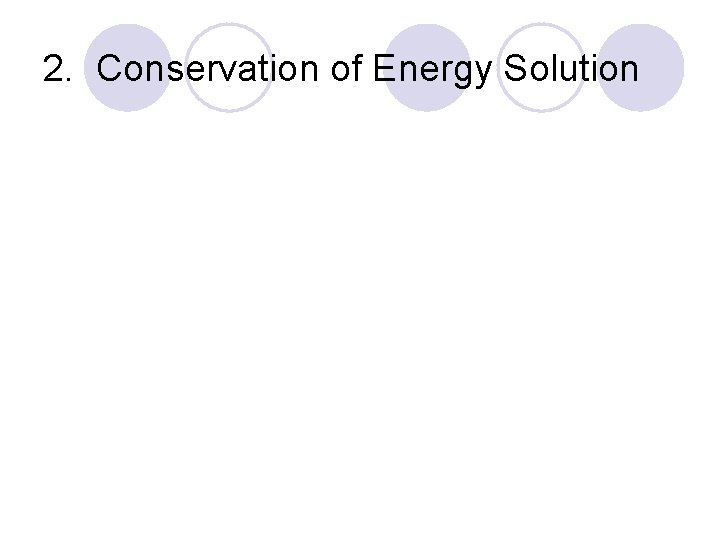2. Conservation of Energy Solutionl If - - - + + + + - The electric field between oppositely charged plates is uniform, except at the ends. a charge is placed between the oppositely charged plates, it will experience a constant force because the field is uniform.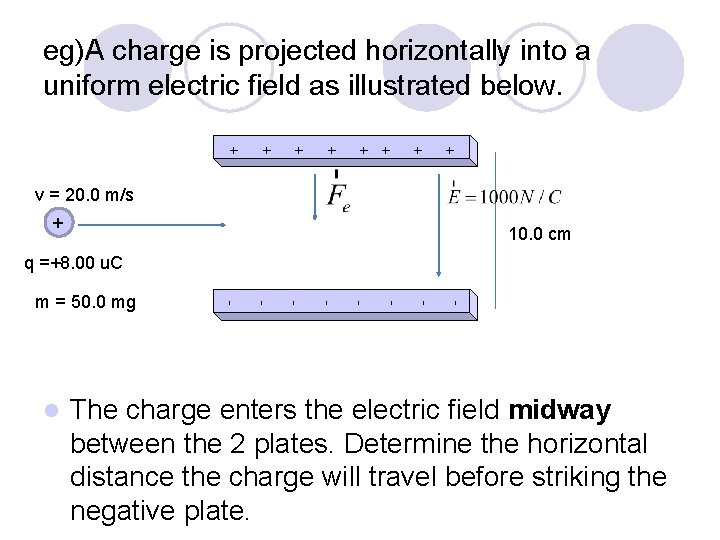eg)A charge is projected horizontally into a uniform electric field as illustrated below. + + + + v = 20. 0 m/s + 10. 0 cm q =+8. 00 u. C - - - - l - m = 50. 0 mg The charge enters the electric field midway between the 2 plates. Determine the horizontal distance the charge will travel before striking the negative plate.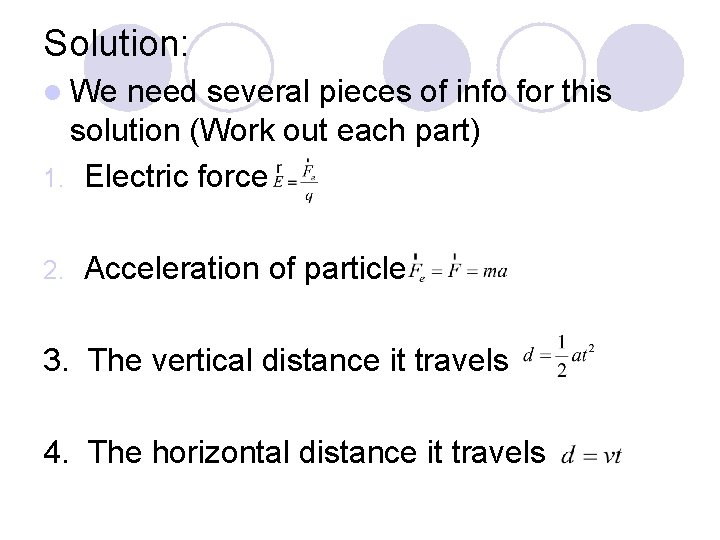Solution: l We need several pieces of info for this solution (Work out each part) 1. Electric force 2. Acceleration of particle 3. The vertical distance it travels 4. The horizontal distance it travels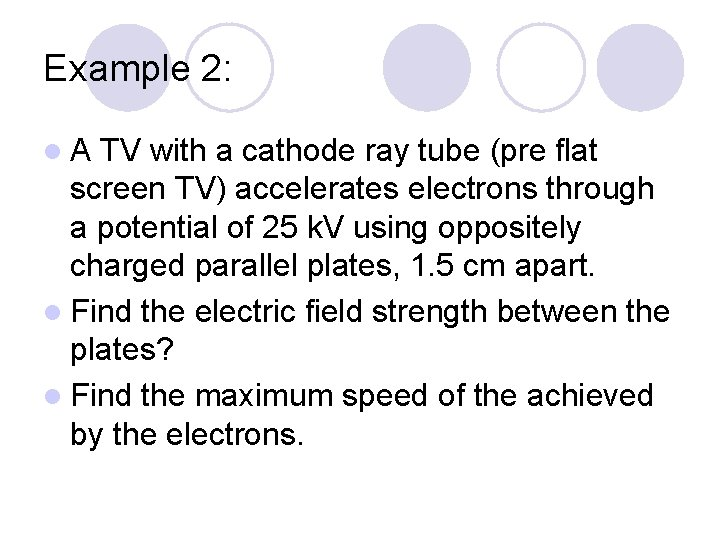Example 2: l. A TV with a cathode ray tube (pre flat screen TV) accelerates electrons through a potential of 25 k. V using oppositely charged parallel plates, 1. 5 cm apart. l Find the electric field strength between the plates? l Find the maximum speed of the achieved by the electrons.Field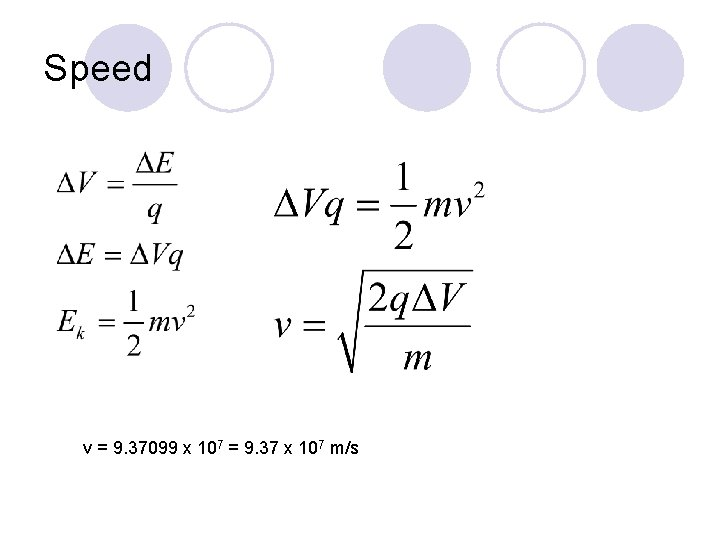Speed v = 9. 37099 x 107 = 9. 37 x 107 m/s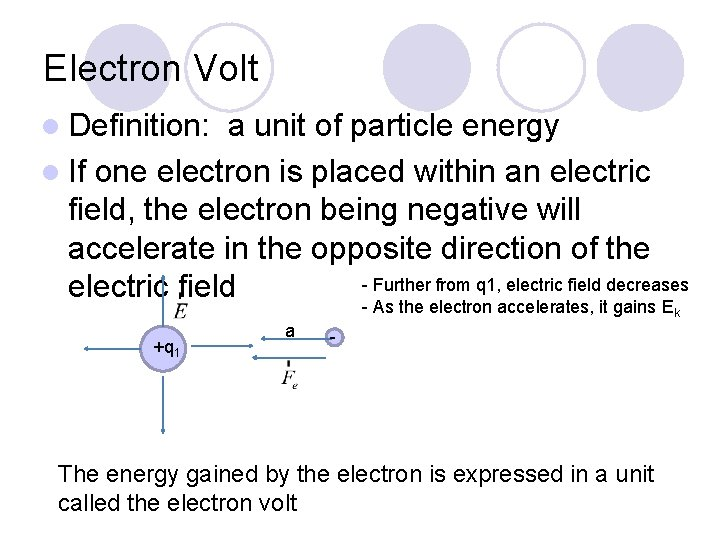Electron Volt l Definition: a unit of particle energy l If one electron is placed within an electric field, the electron being negative will accelerate in the opposite direction of the - Further from q 1, electric field decreases electric field - As the electron accelerates, it gains E k +q 1 a - The energy gained by the electron is expressed in a unit called the electron voltElectron Volt (e. V) – the energy gained by an electron when accelerated through a potential difference of 1. 00 volts. - + - + - +eg) Determine the energy gained in electron volts when a proton accelerates through a potential difference of 120 V. The charge on one electron = 1 e The charge on one proton = 1 e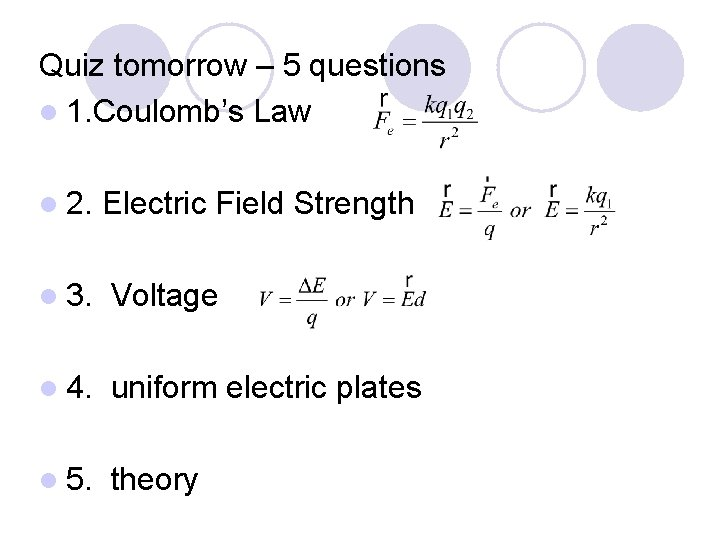Quiz tomorrow – 5 questions l 1. Coulomb’s Law l 2. Electric Field Strength l 3. Voltage l 4. uniform electric plates l 5. theory